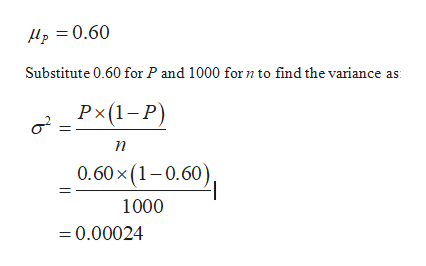# suppose that 60% of all students currently enrolled at all California Community College campuses combined are female. Researchers want to do a survery, and randomly select 1000 students who are currently enrolled at CSU campuses. Let P represent the proportion of students in the sample who are female.h) use binocdf to find the probability between 56% and 64% are female. show calculator input

Question
68 views

suppose that 60% of all students currently enrolled at all California Community College campuses combined are female. Researchers want to do a survery, and randomly select 1000 students who are currently enrolled at CSU campuses. Let P represent the proportion of students in the sample who are female.

h) use binocdf to find the probability between 56% and 64% are female. show calculator input

check_circle

Step 1

Given:

Sample proportion (P) = 0.60%

Sample size (n)=1000

Formula used:

The formula to find the variance is:

Step 2

The formula to find the standard deviation is:

Step 3

Explanation:

Taking a normal approximation as a binomial, the sampling distribution of samp...help_outlineImage TranscriptioncloseHp =0.60 Substitute 0.60 for P and 1000 for n to find the variance Px(1-P) 0.60x (1-0.60), 1000 =0.00024 fullscreen

### Want to see the full answer?

See Solution

#### Want to see this answer and more?

Solutions are written by subject experts who are available 24/7. Questions are typically answered within 1 hour.*

See Solution
*Response times may vary by subject and question.
Tagged in

### Statistics